Index of free lessons | Search free lessons | Print this page | Local tutors

Computational Tricks: Example 2 (page 2 of 2)

• Find all the zeroes of 6x4 – 13x3 – 34x2 + 80x – 25.

The Rational Roots Test says that the possible zeroes are: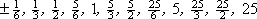It is easy to check if x = –1 or x = 1 is a zero: plug them in! You will be multiplying the coefficients by –1 or by 1, according to the x-value and the exponent, which is simple enough:

f(–1) = 6 + 13 – 34 – 80 – 25 = –120

f(1) = 6 – 13 – 34 + 80 – 25 = 14

So neither x = –1 nor x = 1 is a zero, but, because of the sign change between f(–1) = –120 and f(1) = 14, there must be a zero in between x = –1 and x = 1. However, I'd rather avoid fractions if at all possible, so I'm going to try the next biggest non-fractional possible zeroes, being x = –5 and x = 5.

First I'll try x = –5: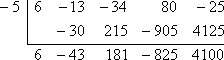So x = –5 is not a zero. However,  f (–5) = 4100 is positive and  f (–1) = –120 is negative, so there must be a zero in between x = –5 and x = –1. And since  f (–1) = –120 is "closer" to zero than is f (–5) = 4100, I would guess that the zero is "closer" to x = –1 than to x = –5. Also, I can see that there is no need to test zeroes below x = –5, because I divided by a negative, and the bottom row has alternating signs.

Let's see if x = 5 is a zero: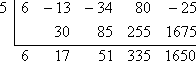So x = 5 is not a zero.  Also,  f(5) = 1650 and f(1) = 14 are positive, so I can't tell if there is a zero in between x = 1 and x = 5.

Descartes' Rule of Signs says that f(x) = 6x4 – 13x3 – 34x2 + 80x – 25 has three or one positive zeroes (so we are assured of at least one positive zero!) and one negative zero. So there are either two real-number zeroes (so the other solutions of this fourth-degree polynomial are complex) or four real-number zeroes. Since there is only one negative root and possibly as many as three positive roots, it looks to me as though my odds of finding a rational zero are better if I try the positive side.

Since f(–1) = –120 is negative and f(1) = 14 is positive, then there must be a zero between x = –1 and x = 1. I'll try x = 1/2: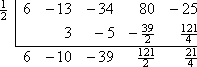Nope. But the remainder says that   f( 1/2) = 21/4, which is a positive number. So I know, from the sign change, that there must be a zero between x = –1 and x = 1/2. I'll try x = 1/3: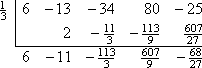This isn't a root either. Also, since the remainder says that f( 1/3) = –68/27, the zero must be between x = 1/3 and x = 1/2. However, there are no rational candidates between x = 1/3 and x = 1/2, so the zero must be irrational. I'll have to look elsewhere for a rational zero.

I have better odds of finding positive zeroes than negative ones (from Descartes' Rule of Signs), so I will try to find a zero between x = 1 and x = 5. I'll try x = 5/2: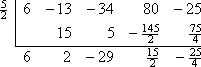Still not a zero. But this remainder says that f( 5/2) = –25/4, which is negative, and I already knew that f(1) = 14 was positive. This means that now I know that there is a zero between x = 1 and x = 5/2. So I'll try x = 5/3: Copyright © Elizabeth Stapel 2002-2011 All Rights Reserved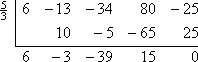I've finally found a zero! Yay!

I still have to find at least one more zero, so I can reduce the polynomial to a quadratic, but now I only have to find the zeroes of the result of the last division, 6x3 – 3x2 – 39x + 15. That is, I am trying to solve 6x3 – 3x2 – 39x + 15 = 0. Why not make life easier on myself, since everything happens to be a multiple of 3, and divide off the 3? This leaves me with 2x3x2 – 13x + 5 = 0. This also reduces the number of possible rational zeroes; my list now consists only of: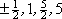I have already eliminated –5, 5, –1, 1, –1/2, and 1/2 from my list of possible zeroes. Combining these earlier deletions with my new shorter list leaves me with only –5/2 and 5/2 to try. This polynomial has been messy so far, so I'll try the negative value: x = –5/2: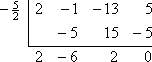And there's my other rational zero. Finally!

Now I'm left with just a simple quadratic, which I can solve with the Quadratic Formula:

2x2 – 6x + 2 = 0

x2 – 3x + 1 = 0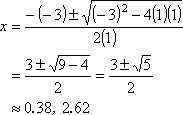Then the zeroes are: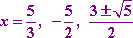Phew! That was painful! But be assured: I did not "go out of my way" to make these examples difficult. They are actually fairly representative, and the steps shown in these examples display the path I actually took (with pencil and paper) to try to find the zeroes of these polynomial. There is no magic "trick" to these exercises, there is no algorithm, there is no "secret". They just take time. So give yourself some time, grab some scratch paper, and practice, practice, practice!

Advisory: In "real life", there is absolutely no guarantee that a given polynomial will have a rational root. As a matter of fact, there probably won't be. In these cases, using graphing software or some other numerical method is the only practical way to approximate the zeroes. However, in an algebra class, when they give you this type of problem, you can generally rest assured that there will be enough rational roots to let you get down to a quadratic. (That is, when they tell you to find the zeroes, they will have given you zeroes that are actually find-able.) You should not be surprised, however, when the final quadratic spits out irrational or complex-valued stink-nasties.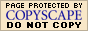WyzAnt Tutoring